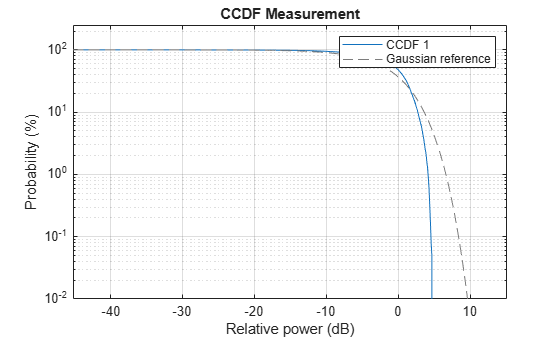# plotCCDF

Plot CCDF curves

Since R2022a

## Syntax

``H = plotCCDF(meter)``
``H = plotCCDF(meter,ax)``
``H = plotCCDF(meter,GaussianReference=true)``
``H = plotCCDF(___,Name=Value)``

## Description

````H = plotCCDF(meter)` plots CCDF measurements in the `powermeter` System object™ `meter` and returns the plot handles as an output `H`. The number of curves plotted equals the number of input channels.```
````H = plotCCDF(meter,ax)` plots CCDF measurements using the `axes` object `axis`.```

example

````H = plotCCDF(meter,GaussianReference=true)`, uses the optional name-value argument `GaussianReference`=`true` to add a Gaussian reference curve, representing the power of a complex white Gaussian noise calculated as a chi-squared distribution, on the CCDF plot. By default `GaussianReference`=`false`.```
````H = plotCCDF(___,Name=Value)`, additionally you can use the name-value arguments of the `plot` function to customize the configuration of the CCDF plot. For example, plotCCDF(meter,LineWidth=2) plots the CCDF curves with a line width of 2 points.```

## Examples

collapse all

Compute the average power measurement of the generated voltage signal.

```x = complex(rand(10000,1)-0.5,rand(10000,1)-0.5); pm = powermeter(ComputeCCDF=true); averagePower = pm(x); % power in dB```

Compute the probability that the instantaneous power of the signal is 3 dB above the average power.

`prob = probability(pm,3) % probability in percentage`
```prob = 7.6009 ```

Plot the CCDF curve.

`plotCCDF(pm,GaussianReference=true)`Find the relative power for the given probability.

`relativepwr = relativePower(pm,prob)`
```relativepwr = 3.0000 ```

## Input Arguments

collapse all

Power meter, specified as a `powermeter` System object.

Target axes, specified as an `axes` object. If you do not specify the axes, MATLAB plots into the current axes. The x-axis represents the relative power in dB, the ratio of instantaneous signal power to the average signal power. The y-axis represents the probability, in percentage, that the relative power exceeds a specified dB level.

## Output Arguments

collapse all

Plot handle output, returned as a `line` object. For more information about plot handle properties, see Line Properties.

## Version History

Introduced in R2022a